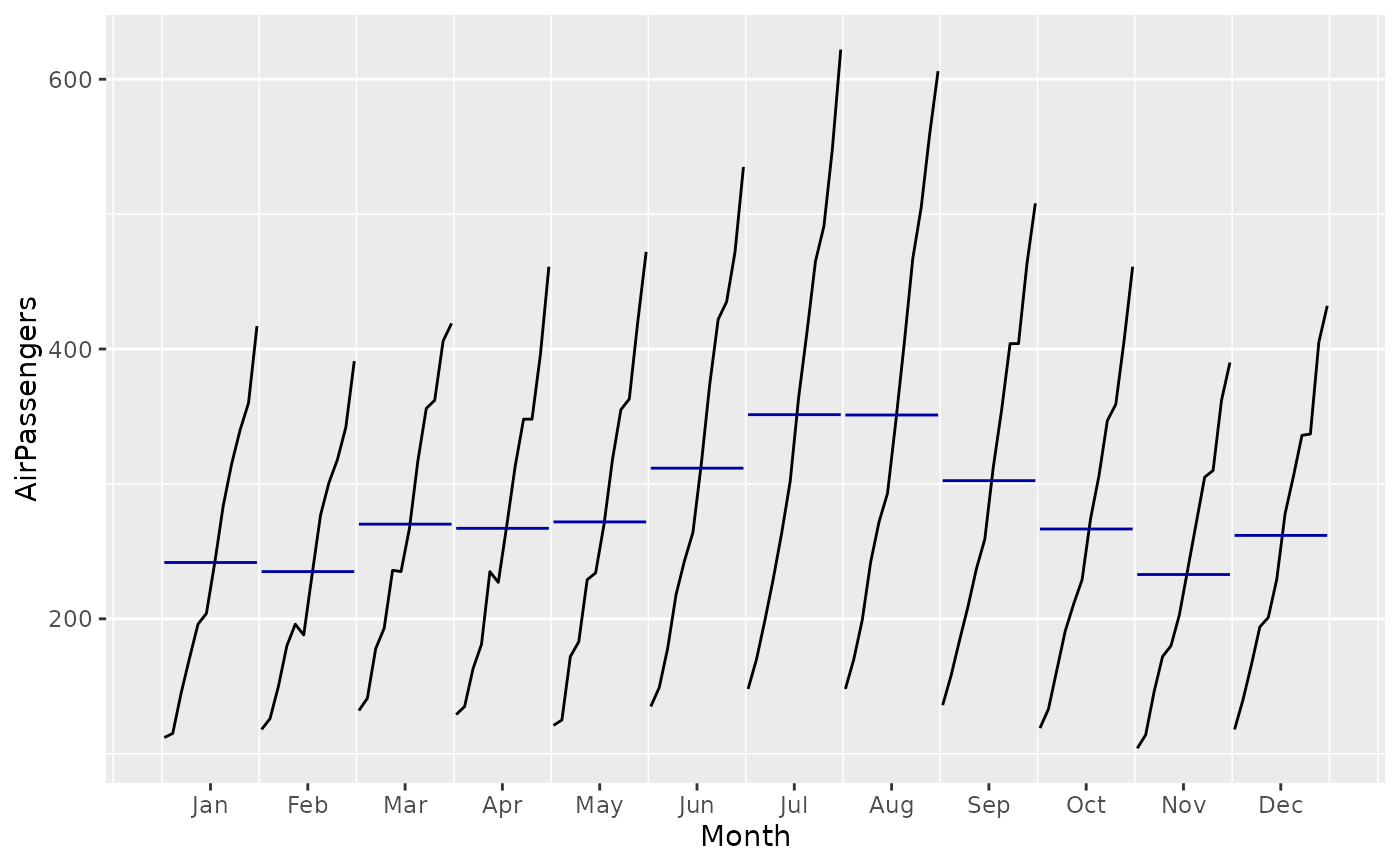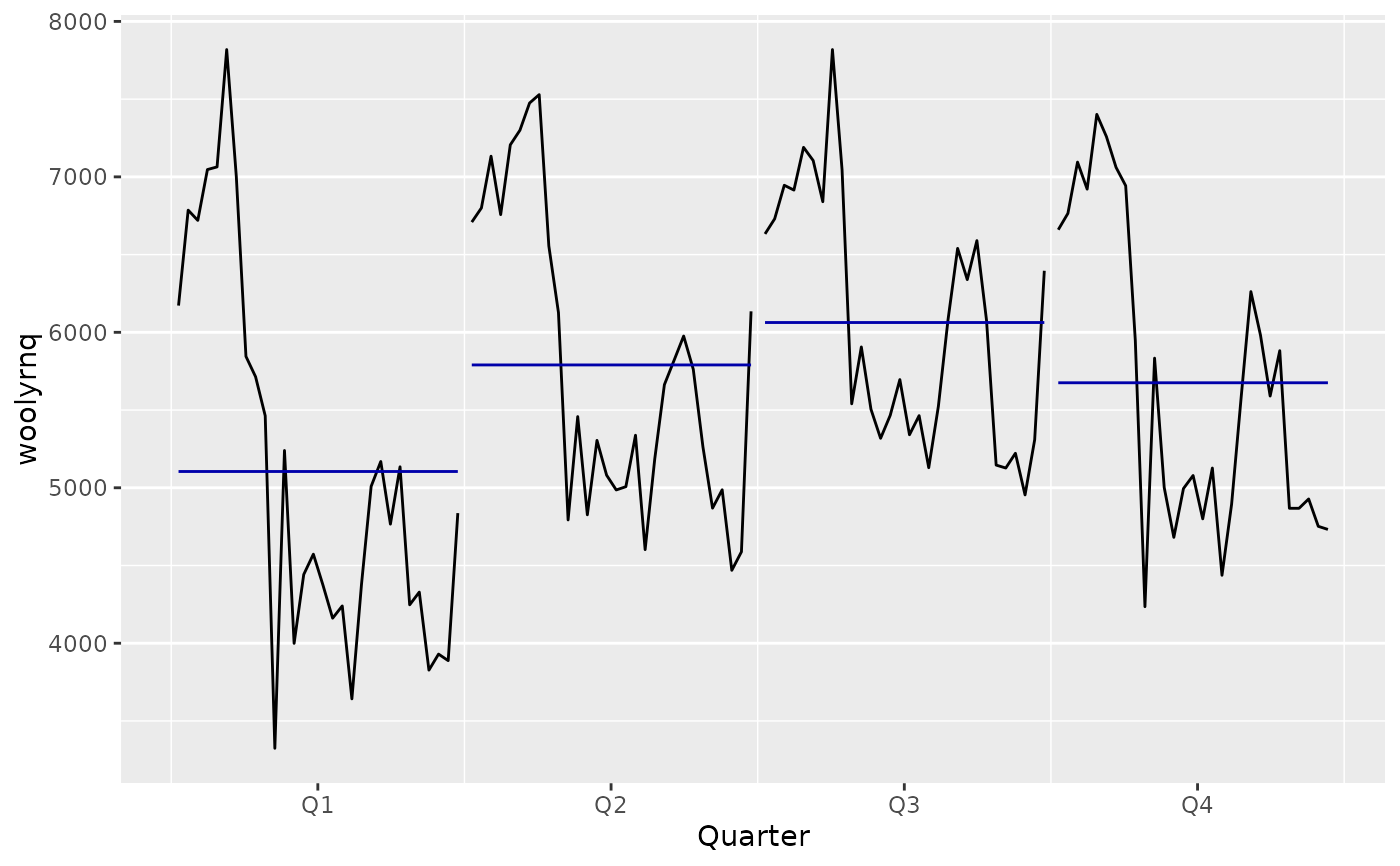Plots a subseries plot using ggplot. Each season is plotted as a separate mini time series. The blue lines represent the mean of the observations within each season.

ggmonthplot(x, labels = NULL, times = time(x), phase = cycle(x), ...)

ggsubseriesplot(x, labels = NULL, times = time(x), phase = cycle(x), ...)

## Arguments

x a time series object (type ts). A vector of labels to use for each 'season' A vector of times for each observation A vector of seasonal components Not used (for consistency with monthplot)

## Value

Returns an object of class ggplot.

## Details

The ggmonthplot function is simply a wrapper for ggsubseriesplot as a convenience for users familiar with monthplot.

monthplot

## Author

Mitchell O'Hara-Wild

## Examples


ggsubseriesplot(AirPassengers)ggsubseriesplot(woolyrnq)